# NCERT Solutions Class 5 Mathematics Chapter 7 Can you see the pattern

## Chapter 7 Can you see the pattern Class 5 Mathematics NCERT Solutions

Class 5 Mathematics students should refer to the following NCERT questions with answers for Chapter 7 Can you see the pattern in Class 5. These NCERT Solutions with answers for Class 5 Mathematics will come in exams and help you to score good marks

### Chapter 7 Can you see the pattern NCERT Solutions Class 5 Mathematics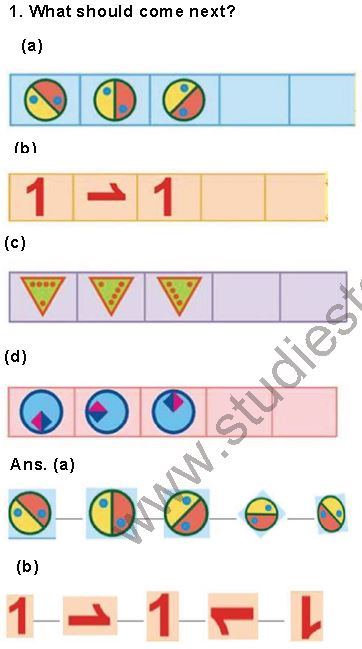3. Magic Squares
____Do you remember magic triangles? Cone now, let’s make some magic squares.
Q. Fill this square using all the numbers from 46 to 54.
Ans.
In this magic square, the sum of each of the row of numbers (across down and diagonally) is always the same. We have to complete the magic squares, remembering that the numbers in each line are equal to 150.
Clearly:
In 3 rd row: The required number = 150 - 52 - 47 = 150 - 99 = 51
In 3 rd column: The required number = 150 - 49 - 47 = 150 - 96 = 54
In 2 nd row: The required number = 150 - 46 - 54 = 150 - 100 = 50
In 2 nd column: The required number = 150 - 50 - 52 = 150 - 102 = 188
In 1 st row: The required number = 150 - 18 - 49 = 150 - 97 = 53
Therefore, the complete magic square isQ. Fill this square suing all the numbers from 21 to 29.
Rule: The total of each side is 75.
Ans.
Let us fix 26 on the top most left hand side box.
Taking the diagonal of the square, we have
26 + 25 = 51 and 75 - 51 = 24
Therefore, put 24 at the end of this diagonal.
Fix 22 on the top most - right side box.
Taking the diagonal in which 22 lies, we have
22 + 25 = 47 and 75 - 47 = 28
Therefore, put 28 at the end of this diagonal.
Clearly,
In 1 st row: The required number = 75 - (26 + 22) = 75 - 48 = 27
In 1 st column: The required number = 75 - (26 + 28) = 75 - 54 = 21
In 2 nd row: The required number = 75 - (21 + 25) = 75 - 46 = 29
In 2 nd column: The required number = 75 - (28 + 24) = 75 - 52 = 27
Therefore, the complete magic square is as shown below: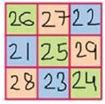3. Fill in the blank spaces in the same way.
(a) 14 +…..+……= 34 + 24 + 20
(b) ……+ 42 + ……= 65 +…. + 80
(c) 200 + 300 + ….. = ….. + 400 + …….
(d) ….. + ….. + ….. = ….. + ….. + …….
Ans.

(a) 14 + 20 + 34 = 34 + 14 +20
(b) 80 + 42 + 65 = 65 + 42 + 80
(c) 200 + 300 + 400 = 300 + 400 + 200
(d) 34 + 29 +47 = 47 + 34 +29

4. Now you try and change these numbers into special numbers:
(a) 28 (b) 132 (c) 273
Ans. (a)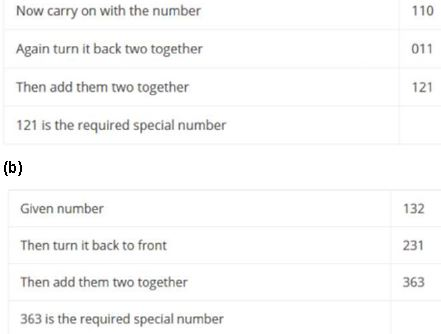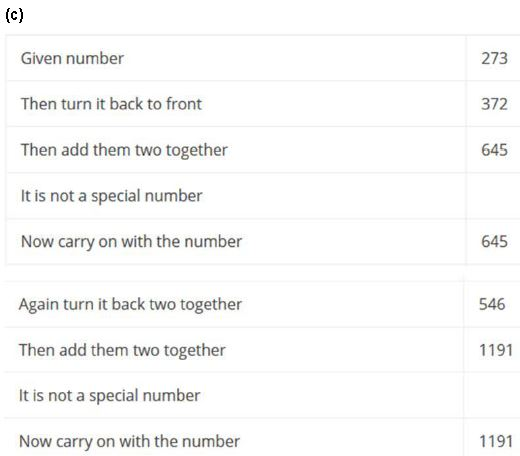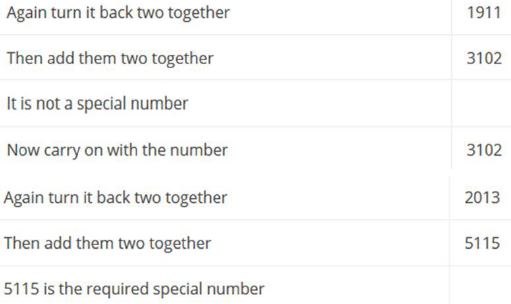5. Choose any 3 x 3 box from a calendar and find the total in the same way.
Play this game with your family.
Ans.
Let us mark a 3 3 box (9 dates) on the calendar and see some magic.Take the smallest number: 2
10
Multiply it by 9 x 9
Total 90

6. Take any number. Now multiply it by 2, 3, …… at every step. Also add 3 to it at each step. Look at the difference in the answer. Is it the same at every step?
12 2 + 3 = 27
12 3 + 3 = 39
12 4 + 3 = 51
12 5 + 3 = 63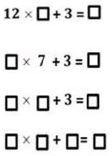Ans. Filling in the blank boxes, we have
12 6 + 3 = 75
12 7 + 3 = 87
12 8 + 3 = 99
12 9 + 3 = 111

7. Look at the numbers below. Look for the pattern. Can you take it forward?
(9 – 1) ÷ 8 = 1
(98 – 2) ÷ 8 = 12
(987 – 3) ÷ 8 = 123
(9876 – 4) ÷ 8 = ____
(98765 – 5) ÷ 8 = ____
( ____–__ ) ÷ 8 = ____
( ____–__ ) ÷ 8 = ____
Ans.
Yes, the given pattern can be taken forward as under:
(9 – 1) ÷ 8 = 1
(98 – 2) ÷ 8 = 12
(987 – 3) ÷ 8 = 123
(9876 – 4) ÷ 8 = 1234
(98765 – 5) ÷ 8 = 12345
(987654 – 6) ÷ 8 = 123456
(9876543 – 7) ÷8 = 1234567

1 + 2 + 3 + 4 + 5 + 6 + 7 + 8 + 9 + 10 = 55
11 + 12 + .. + .. + .. + .. + .. + .. + .. + 20 = 155
21 + .. + .. + .. + .. + .. + .. + .. + .. + 30 = …
31 + .. + .. + .. + .. + .. + .. + .. + .. + 40 = …
41 + .. + .. + .. + .. + .. + .. + .. + .. + 50 = …
51 + .. + .. + .. + .. + .. + .. + .. + .. + 60 = 555
61 + .. + .. + .. + .. + .. + .. + .. + .. + 70 = …
Ans. 1 + 2 + 3 + 4 + 5 + 6 + 7 + 8 + 9 + 10 = 55
11 + 12 + 13 + 14 + 15 + 16 + 17 + 18 + 19 + 20 = 155
21 + 22 + 23 + 24 + 25 + 26 + 27 + 28 + 29 + 30 = 255
31 + 32 + 33 + 34 + 35 + 36 + 37 + 38 + 39 + 40 = 355
41 + 42 + 43 + 44 + 45 + 46 + 47 + 48 + 49 + 50 = 455
51 + 52 + 53 + 54 + 55 + 56 + 57 + 58 + 59 + 60 = 555
61 + 62 + 63 + 64 + 65 + 66 + 67 + 68 + 69 + 70 = 655

9. Take the first two odd numbers, now add the, see what you get.
Now, at every step, add the next odd number.
1 + 3 = 4 = 2 x 2
1 + 3 + 5 = 9 = 3 x 3
1 + 3 + 5 + 7 = 16 = 4 x 4How far can you go on?
Ans. Let us complete it.
1 + 3 + 5 + 7 +9 = 25 = 5 5
1 + 3 + 5 + 7 + 9 + 11 = 36 = 6 6
1 + 3 + 5 + 7 + 9 + 11 + 13 = 49 = 7 7

10. Secret Numbers
Banno and binod were playing a guessing game by writing clues about a secret number. Each tried by writing clues about a secret number. Each tried to guess the other’s secret number from the clues. Can you guess their secret numbers?

(a) It is larger than half of 100.
Ans. (a) It is larger than half of 100 means > 50.

(b) It is more than 6 tens and less than 7 tens.
Ans. (b) It is more than 6 tens and less than 7 tens it lies between 60 and 70.

(c) The tens digit is one more than he one’s digit.
Ans. (c) The tens digit is one more than one’s digit is 6-5 =5.

(d) Together the digits have a sum of 11.
Ans. (d) Together the digits have the sum of 11, so the number is 65.

11. Write a set of clues for a secret number of your own. Then give it to a friend to guess your secret answer.
Ans. A set of clues to find secret numbers are:
____ It is larger than half of 100.
It is more than 7 tens and less than 8 tens.
The tens digit is one less than the one’s digit.
Together the digits have a sum of 15.

12. (a) Ask your friend-Write down his age. Add 5 to it. Multiply the sum by 2.
Subtract 10 from it. Next divide it by 2. What do you get?
Ans. (a) Age: 7
Add 5 to it: 7 + 5 = 12
Multiply the sum by 2 = 12 x 2 = 24
Subtract 10 from it = 24 - 10 = 14
Divide it by 2 =14/2 = 7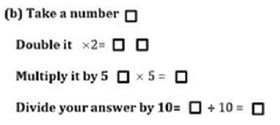Ans.
(b) Take a number as 5(say)
Double it 5 x 2 = 10
Multiply by 5 = 10 x 5 = 50
Thus, we got the supposed answer.

(c) Look at this pattern of number and take it forward.
1 = 1 x 1
121 = 11 x 11
12321 = 111 x 111
1234321 = ?
Ans.
(c) Taking the pattern forward, we have
1234321 = 1111 x 1111.

 NCERT Solutions Class 5 Mathematics Chapter 1 The Fish Tale
 NCERT Solutions Class 5 Mathematics Chapter 2 Shapes and Angles
 NCERT Solutions Class 5 Mathematics Chapter 3 How Many Squares
 NCERT Solutions Class 5 Mathematics Chapter 4 Parts and Wholes
 NCERT Solutions Class 5 Mathematics Chapter 5 Does it look the same
 NCERT Solutions Class 5 Mathematics Chapter 6 Be my multiply
 NCERT Solutions Class 5 Mathematics Chapter 7 Can you see the pattern
 NCERT Solutions Class 5 Mathematics Chapter 8 Mapping Your Way
 NCERT Solutions Class 5 Mathematics Chapter 9 Boxes And Sketches
 NCERT Solutions Class 5 Mathematics Chapter 10 Tenths And Hundredths
 NCERT Solutions Class 5 Mathematics Chapter 11 Area and its Boundary
 NCERT Solutions Class 5 Mathematics Chapter 12 Smart Charts
 NCERT Solutions Class 5 Mathematics Chapter 13 Ways of Multiply and Divide
 NCERT Solutions Class 5 Mathematics Chapter 14 How Big How Heavy

## More Study Material

Tags

### NCERT Solutions Class 5 Mathematics Chapter 7 Can you see the pattern

NCERT Solutions Class 5 Mathematics Chapter 7 Can you see the pattern is available on our website www.studiestoday.com for free download in Pdf. You can read the solutions to all questions given in your Class 5 Mathematics textbook online or you can easily download them in pdf.

### Chapter 7 Can you see the pattern Class 5 Mathematics NCERT Solutions

The Class 5 Mathematics NCERT Solutions Chapter 7 Can you see the pattern are designed in a way that will help to improve the overall understanding of students. The answers to each question in Chapter 7 Can you see the pattern of Mathematics Class 5 has been designed based on the latest syllabus released for the current year. We have also provided detailed explanations for all difficult topics in Chapter 7 Can you see the pattern Class 5 chapter of Mathematics so that it can be easier for students to understand all answers.

#### NCERT Solutions Chapter 7 Can you see the pattern Class 5 Mathematics

Class 5 Mathematics NCERT Solutions Chapter 7 Can you see the pattern is a really good source using which the students can get more marks in exams. The same questions will be coming in your Class 5 Mathematics exam. Learn the Chapter 7 Can you see the pattern questions and answers daily to get a higher score. Chapter 7 Can you see the pattern of your Mathematics textbook has a lot of questions at the end of chapter to test the students understanding of the concepts taught in the chapter. Students have to solve the questions and refer to the step-by-step solutions provided by Mathematics teachers on studiestoday to get better problem-solving skills.

#### Chapter 7 Can you see the pattern Class 5 NCERT Solution Mathematics

These solutions of Chapter 7 Can you see the pattern NCERT Questions given in your textbook for Class 5 Mathematics have been designed to help students understand the difficult topics of Mathematics in an easy manner. These will also help to build a strong foundation in the Mathematics. There is a combination of theoretical and practical questions relating to all chapters in Mathematics to check the overall learning of the students of Class 5.

#### Class 5 NCERT Solution Mathematics Chapter 7 Can you see the pattern

NCERT Solutions Class 5 Mathematics Chapter 7 Can you see the pattern detailed answers are given with the objective of helping students compare their answers with the example. NCERT solutions for Class 5 Mathematics provide a strong foundation for every chapter. They ensure a smooth and easy knowledge of Revision notes for Class 5 Mathematics. As suggested by the HRD ministry, they will perform a major role in JEE. Students can easily download these solutions and use them to prepare for upcoming exams and also go through the Question Papers for Class 5 Mathematics to clarify all doubts

Where can I download latest NCERT Solutions for Class 5 Mathematics Chapter 7 Can you see the pattern

You can download the NCERT Solutions for Class 5 Mathematics Chapter 7 Can you see the pattern for latest session from StudiesToday.com

Can I download the NCERT Solutions of Class 5 Mathematics Chapter 7 Can you see the pattern in Pdf

Yes, you can click on the link above and download NCERT Solutions in PDFs for Class 5 for Mathematics Chapter 7 Can you see the pattern

Are the Class 5 Mathematics Chapter 7 Can you see the pattern NCERT Solutions available for the latest session

Yes, the NCERT Solutions issued for Class 5 Mathematics Chapter 7 Can you see the pattern have been made available here for latest academic session

How can I download the Chapter 7 Can you see the pattern Class 5 Mathematics NCERT Solutions

You can easily access the links above and download the Chapter 7 Can you see the pattern Class 5 NCERT Solutions Mathematics for each chapter

Is there any charge for the NCERT Solutions for Class 5 Mathematics Chapter 7 Can you see the pattern

There is no charge for the NCERT Solutions for Class 5 Mathematics Chapter 7 Can you see the pattern you can download everything free

How can I improve my scores by reading NCERT Solutions in Class 5 Mathematics Chapter 7 Can you see the pattern

Regular revision of NCERT Solutions given on studiestoday for Class 5 subject Mathematics Chapter 7 Can you see the pattern can help you to score better marks in exams

Are there any websites that offer free NCERT solutions for Chapter 7 Can you see the pattern Class 5 Mathematics

Yes, studiestoday.com provides all latest NCERT Chapter 7 Can you see the pattern Class 5 Mathematics solutions based on the latest books for the current academic session

Can NCERT solutions for Class 5 Mathematics Chapter 7 Can you see the pattern be accessed on mobile devices

Yes, studiestoday provides NCERT solutions for Chapter 7 Can you see the pattern Class 5 Mathematics in mobile-friendly format and can be accessed on smartphones and tablets.

Are NCERT solutions for Class 5 Chapter 7 Can you see the pattern Mathematics available in multiple languages

Yes, NCERT solutions for Class 5 Chapter 7 Can you see the pattern Mathematics are available in multiple languages, including English, Hindi